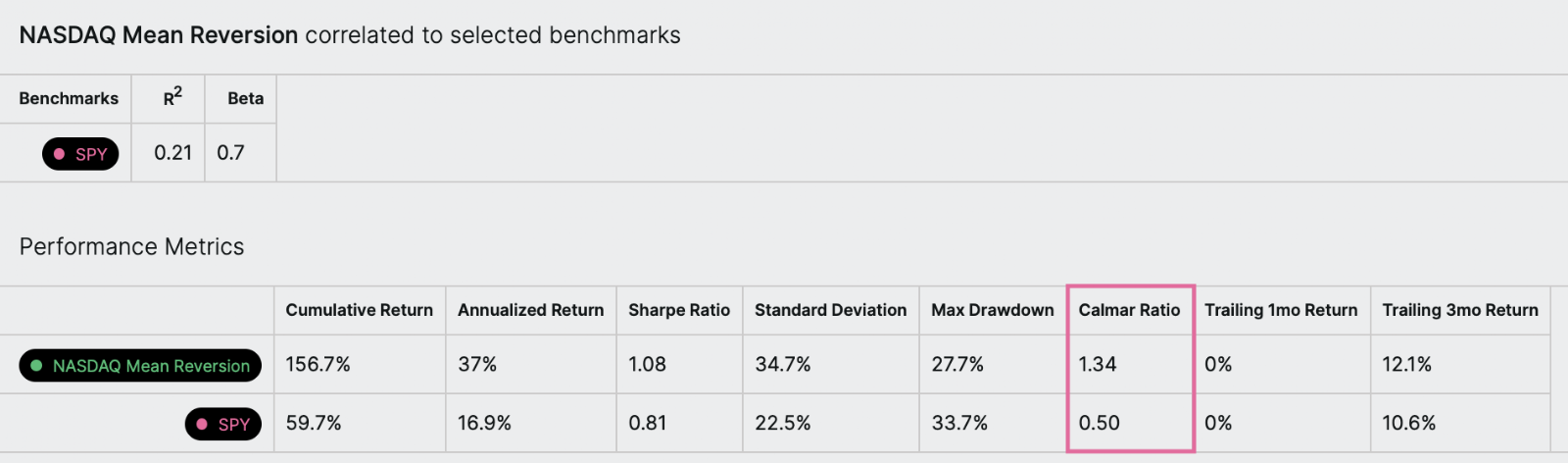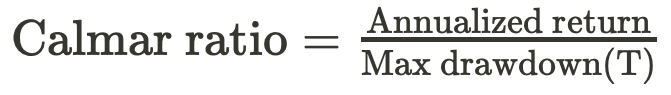# Calmar ratio

What is the Calmar ratio?

The Calmar ratio, also called the drawdown ratio, is a measure of risk-adjusted return. It was developed by Terry Young, who owned the investment management company California Managed Accounts. Calmar is an acronym for CALifornia Managed Accounts Reports, the name of the company’s newsletter.

The Calmar ratio is an investment’s annualized rate of return divided by its max drawdown for a selected time period.

An example

Let’s take a look at an example. In this backtest, we see the symphony’s Calmar ratio is 1.34 while the benchmark’s (\$SPY) is 0.50. This means that the symphony had a better risk-adjusted return than the benchmark over the backtest time period.What is the formula?What is the step-by-step calculation?

Let's go through the calculation of the Calmar ratio step-by-step for a symphony backtest. Here's how we do it:

Calculate the annualized rate of return.

• Compute the cumulative percent return of the symphony for the time between the first and last date in the backtest.
• Add one to that value.
• Raise that result to the (252/N) power, where N is the number of trading days in the backtest.
• Subtract 1 from that result.

Calculate the maximum drawdown. For the maximum drawdown, we'll look at each value in the backtest period, and keep track of two quantities: the maximum value we've seen and the maximum drawdown we've seen, up to that point.

• Start with both the maximum value and the maximum drawdown value set to 0, and consider each day's value in chronological order.
• If a value is greater than the maximum value we've seen so far, record it as such.
• If not, compute the magnitude of the percentage loss between the maximum value we've seen so far, and the value we are observing; this is the drawdown.
• If this drawdown is greater than the maximum drawdown we've seen so far, record it as such.
• Repeat the above steps until we've observed every value.
• The maximum drawdown we've recorded when we've finished observing all the values is the maximum drawdown over the period.

Divide the annualized rate of return by the maximum drawdown.